# AP Physics 1 Question 411: Answer and Explanation

### Test Information

Question: 411

9. What is the difference between the wavelengths of the 3rd and 4th harmonics of a standing wave fixed at both ends?

• A.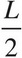• B.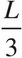• C.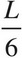• D.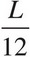The harmonic wavelengths of standing waves fixed at both ends are given by the equation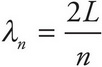. The wavelength of the third harmonic then is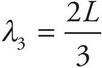, and the wavelength of the fourth harmonic is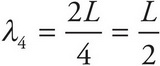. The difference between the wavelengths of these two harmonics is equal to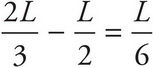.